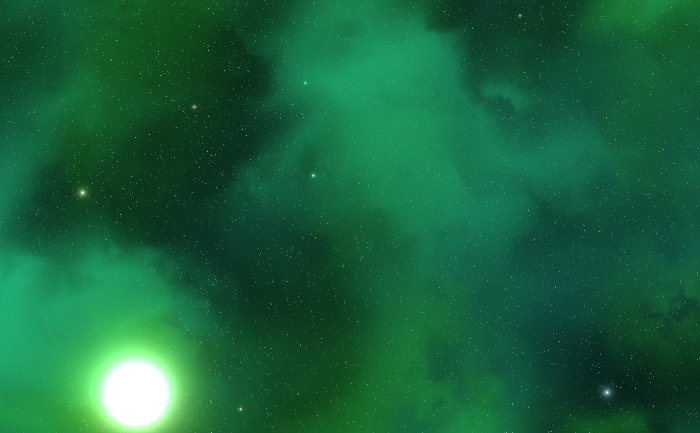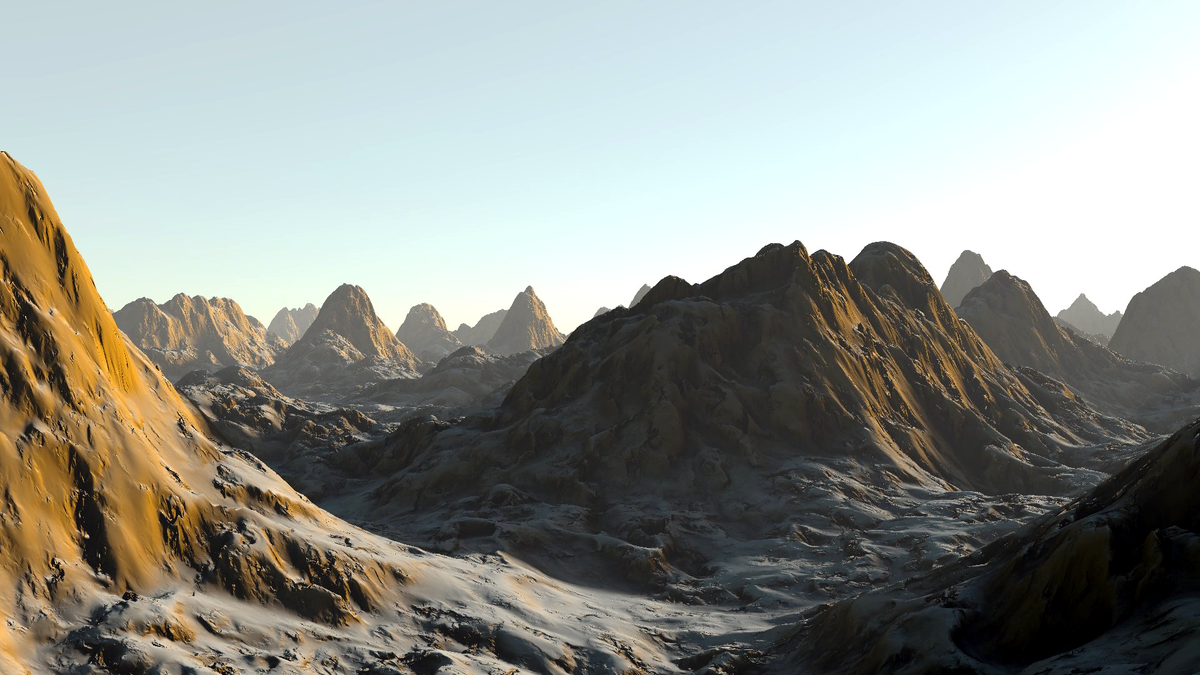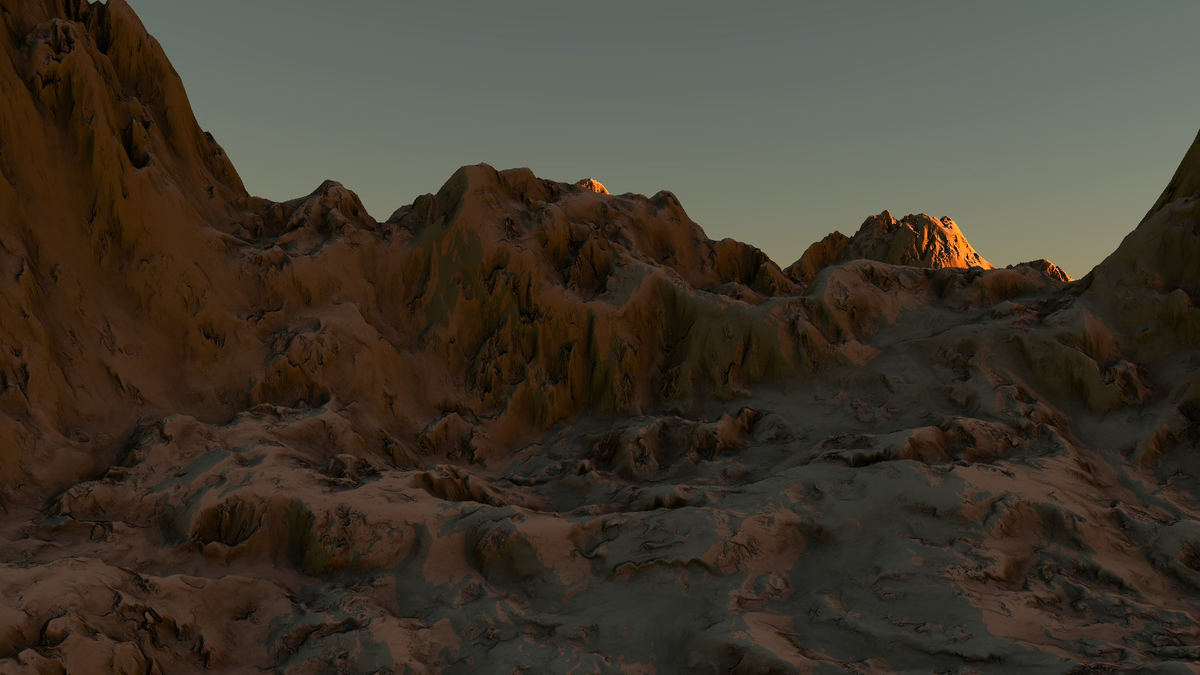# Cursive Noise

Rye Terrell
April 03, 2017

Here’s some example results of a noise function I cooked up that seems to have pretty good variety:In a nutshell, use perlin noise to index into perlin noise, hence the name “cursive” (as in, recursive). It also looks a bit swirly when you zoom in on it, so cursive seems to fit there, too. I wouldn’t believe I was the first to dream this up if all the world told me so, so I’ll just apply that name to my implementation:

float normalnoise(vec2 p) {
return 0.5 + 0.5 * perlin2D(p);
}

float cursive(vec2 p) {
const int steps = 13;
float sigma = 0.7;
float gamma = pow(1.0/sigma, float(steps));
vec2 displace = vec2(0);
for (int i = 0; i < steps; i++) {
displace = 1.5 * vec2(
normalnoise(p.xy * gamma + displace),
normalnoise(p.yx * gamma + displace)
);
gamma *= sigma;
}
return normalnoise(p * gamma + displace);
}


You can see this noise function in action in my badlands terrain generator and my 2D space scene generator.

And Bob’s your uncle. Have fun, and if you make something cool with it, let me know!